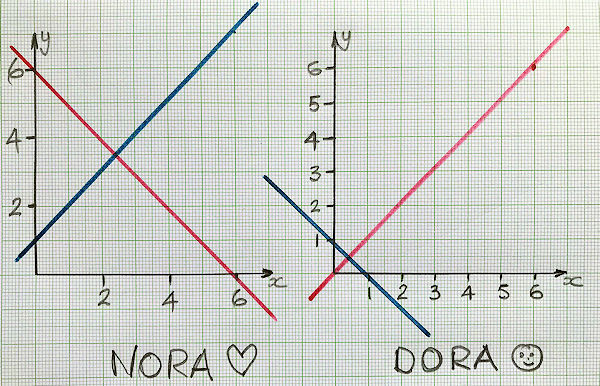# Exam-Style Questions on Simultaneous Equations

## Problems on Simultaneous Equations adapted from questions set in previous Mathematics exams.

### 1.

IGCSE Core

Nora and Dora are trying to solve the following simultaneous equations by finding the intersection of their graphs.

$$y+x=6$$ $$y-x=1$$

Here are their graphs:(a) Who has plotted the graphs most correctly?

(b) What is the correct solution?

### 2.

GCSE Higher

Solve the simultaneous equations

$$5x+2y=0$$ $$5x-3y=15$$

### 3.

GCSE Higher

Estimate the solutions of the following simultaneous equations using their graphs as drawn on the grid below.

$$3x-5y=17$$ $$y=5-\frac{7x}{5}$$### 4.

GCSE Higher

Solve the simultaneous equations:

$$3x-5y=18$$ $$4x+2y=11$$

### 5.

GCSE Higher

A rectangular sheet of paper can be cut into two identical rectangular pieces in two different ways, either by cutting along line A or by cutting along line B.When the original sheet of paper is cut along line A, the perimeter of each of the two pieces is 56 cm.

When the original sheet of paper is cut along line B, the perimeter of each of the two pieces is 64 cm.

What is the perimeter of the original sheet of paper?

### 6.

GCSE Higher

Draw the graph of $$y = 2x^2 + 3x - 7$$ for $$-3.5 \le x \le 2$$. Draw suitable straight lines to find approximate solutions (to one decimal place) of the equations:

(a) $$2x^2 +3x - 9 = 0$$

(b) $$2x^2 +4x - 7 = 0$$

(c) $$2x^2 - x -5 = 3 - x$$

### 7.

GCSE Higher

Solve algebraically the simultaneous equations to find the solution where $$-10 \le x \le 10$$.

$$3x^2 - y^2 = 11$$ $$5x + 3y = 27$$

### 8.

GCSE Higher

Two numbers are chosen so that the sum of their squares is 25.

If those numbers are represented by $$x$$ and $$y$$ they will also satisfy the equation:

$$y-3x=13$$

Use an algebraic method to find two possible values of $$x$$ and $$y$$ .

### 9.

GCSE Higher

The prices of two watches are in the ratio $$a:b$$.

When the prices are both increased by £10, the ratio becomes $$5 : 7$$.

When the prices are both reduced by £10, the ratio becomes $$1 : 3$$.

Express the ratio $$a:b$$ in its lowest terms.

If you would like space on the right of the question to write out the solution try this Thinning Feature. It will collapse the text into the left half of your screen but large diagrams will remain unchanged.

The exam-style questions appearing on this site are based on those set in previous examinations (or sample assessment papers for future examinations) by the major examination boards. The wording, diagrams and figures used in these questions have been changed from the originals so that students can have fresh, relevant problem solving practice even if they have previously worked through the related exam paper.

The solutions to the questions on this website are only available to those who have a Transum Subscription.

Exam-Style Questions Main Page

Search for exam-style questions containing a particular word or phrase:

To search the entire Transum website use the search box in the grey area below.